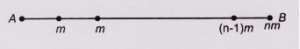Home » Physics » System of particles practice problems with solutions for class 11

# System of particles practice problems with solutions for class 11

This article contains System of particles practice problems with solutions for class 11. In this article I have given numerical problems to solve.
Center of mass : System of particles and Collision
Other assignments(Included with Linear momentum) for this chapter which you can have a look are
Assignment 1
Assignment 2
Assignment 3
Assignment 4


## System of particles practice problems

Question 1 Can the center of mass of a body lie outside the body? If so give examples.

Question 2  AB is a light rod of length n cm. To the rod masses m, 2m, 3m,… nm are attached at distances 1, 2, 3,………, n cm respectively from A. Find the distance from A of the center of mass of rod.

Question 3 Three particles of masses 2 kg, 5 kg and 3 kg are situated at points with position vectors $(\hat i + 4\hat j – 7\hat k) m$, $(3 \hat i – 2\hat j + \hat k) m$ and $(\hat i – 6\hat j + 13\hat k) m$ respectively. Find the position vector of center of mass.

Question 4  Show that the center of mass of a rod of mass M and length L lies midway between its ends, assuming the rod has a uniform mass per unit length.

Question 5 Suppose a rod is non-uniform in length such that it’s mass per unit length varies linearly with $x$ according to the expression  , where $\alpha$ is a constant. Find the $x$ coordinate of the center of mass as a fraction of $L$.

Question 6 Three balls are thrown into air simultaneously. What is the acceleration of their center of mass while they are in motion?

Question 7 A bomb, initially at rest, explodes into several pieces.

(a) Is linear momentum constant?

(b) Is kinetic energy constant? Explain

Question 8 The mass of the moon is about 0.013 times the mass of earth and the distance from the center of the moon to the center of earth is about 60 times the radius of earth. How far is the center of mass of earth-moon system from the center of earth?

Question 9 A  2.0 .kg  particle  has  a  velocity  $(2.0\hat i – 3.0\hat j)m/s$, and a 3.0 kg  particle  has  a  velocity

$(1.0\hat i + 6.0\hat j)m/s$ .Find

(a) the velocity of the center of mass and

(b) the total momentum of the system.

Question 10 A 2.0 Kg particle has velocity $\vec {v_1}=(2\hat i – 10 t \hat j)$ m/s , where $t$ is in seconds. A 3.0 Kg particle moves with a constant velocity of $\vec {v_2}=(4.0\hat i )$ m/s. At t=0.50 s , find

(a) the velocity of center of mass

(b) the acceleration of center of mass and

(c) the total momentum of the system

## Solutions to above System of particles practice problems

Answer 1 Yes it can be outside the body for example a bangle or a ring as their center of mass is in center of its body which actually is outside the body as a bangle or a ring is hollow.If we consider origin to be at point A , then the distance of center of mass from origin can be written as

$${{X}_{CM}}=\frac{m\cdot 1+2m\cdot 2+3m\cdot 3+……..+nm\cdot n}{m+2m+3m+……..nm}cm$$

$$=\frac{m[{{1}^{2}}+{{2}^{2}}+{{3}^{2}}+……..+{{n}^{2}}]}{m[1+2+3+….+n]}cm$$

$$=\frac{\frac{n(n+1)(2n+1)}{6}}{\frac{n(n+1)}{2}}$$

$$=\frac{2n+1}{3}cm$$

Since, for$i=1,2,3,……$

$$\sum\limits_{i\text{ }=1}^{n}{{{i}^{2}}}=\frac{n\left( n+1 \right)\left( 2n+1 \right)}{6}$$

https://proofwiki.org/wiki/Sum_of_Sequence_of_Squares

and sum of first $n$ natural numbers taken 1 at a time is

$$\sum\limits_{i=1}^{n}{i}=\frac{n(n+1)}{2}$$

Answer 3 $\vec r_{cm} = (2\hat i -2 \hat j +3\hat k)m$

Answer 5 Note that here $\lambda$ is not constant. So we have,

\begin{equation*}

X_{CM}=\frac{1}{M}\int\limits_{0}^{L}{xdm}

\end{equation*}

Here $dm=\lambda dx$

So,

\begin{equation*}

{X_{CM}} = \frac{1}{M}\int\limits_0^L {x\lambda dx = \frac{\alpha }{M}\int\limits_0^L {{x^2}dx} = \frac{{\lambda {L^3}}}{{3M}}}

\end{equation*}

Now we can eliminate $\alpha$ since total mass of the rod is related to $\alpha$ through the relationship

$$M=\int{dm}=\int\limits_{0}^{L}{\lambda dx}=\int\limits_{0}^{L}{\alpha xdx}=\frac{\alpha {{x}^{2}}}{2}$$

Now substitute this value into the expression for $X_{CM}$ gives

$${{X}_{CM}}=\frac{\alpha {{L}^{3}}}{3\alpha {{L}^{2}}/2}=\frac{2L}{3}$$

Answer 6 9.8 m/s² downwards. External force acting on the system is gravity therefore the acceleration of the center of mass would be acceleration due to gravity.

Answer 7 Yes linear momentum is conserved. A massive piece will move with low velocity where as a lighter will move with greater velocity in diametrically opposite directions. So all pieces would have their own respective speed in the right directions and yet the total momentum will become zero as it was before the bomb blast.
But kinetic energy is not conserved. Initially the KE was zero as the bomb was at rest. After explosion all pieces will have KE. And KE cannot be negative and so the total KE of all pieces cannot become zero. Hence no conservation of KE. Of course energy is conserved as the KE of the pieces plus energy produced in the form of light, sound will be exactly equal to the energy given out by the exploding mechanism or material.

Answer 8 It is given in the question that

$m_{M}=0.013m_{E}$

$R_{E}=6400Km$

$X_{M}=60R_{E}$

where $m_M$ is mass of the moon, $R_E$ is the radius of the earth, $X_M$ is the distance from the center of the moon from center of the earth. Note that we are using center of the earth as origin. So we have $X_E=0$

Now we have to first find the position of the center of mass of earth moon system

$$X_{CM}=\frac{m_{E}X_{E}+m_{M}X_{M}} {m_{E}+m_{M}}$$

$$X_{CM}=\frac{m_{E}(0)+0.013m_{E} (60R_{E})} {m_{E}+0.013m_{E}}$$

$$X_{CM}=\frac{0.013(60{{R}_{E}})}{1.013}$$

$$X_{CM}=4928Km$$

Answer 9 (a) $1.4 \hat i+2.4\hat j$ m/sec (b) $(7.0\hat i + 12\hat j)$ Kg –m/s

Answer 10 (a) $3.2\hat i -2\hat j)$m/s

(b) $-4\hat j$ m/s2

(c) $16\hat i -10\hat j$ kg-m/s

Some other resources you can look for reference are
https://en.wikipedia.org/wiki/Center_of_mass
Hope you like this System of particles practice problems with solutions for class 11 and these help you in your exams. If you like this article please share it among your friends.